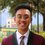# Integral Generalizations

Just keeping a note to self that I can revise and study for my upcoming integration bee. I'd love your advice for studying for it here!

$\displaystyle \int x^ne^x \ dx = n!e^x \left( \frac{x^n}{n!} - \frac{x^{n - 1}}{(n - 1)!} + \frac{x^{n - 2}}{(n - 2)!} - \frac{x^{n - 3}}{(n - 3)!} + \cdots + \right) + C$

\displaystyle \begin{aligned} \int \frac{1}{(x + a)(x + b)} \ dx = \frac{1}{a - b} \ln \left| \frac{x + b}{x + a} \right| + C, & & a > b \end{aligned}

$\displaystyle I_a = \int \frac{1}{x^a + x} \ dx = -\frac{1}{a - 1}\ln \left(1 + \frac{1}{x^{a-1}}\right) + C$

\displaystyle \begin{aligned} I_n = \int_0^{\frac{\pi}{2}} \sin ^n (x) \ dx = \int_0^{\frac{\pi}{2}} \cos ^n (x) \ dx, & & n \geq 3 \end{aligned}

• $\displaystyle I_{2k} = \frac 12 \times \frac 34 \times \frac 56 \times \cdots \times \frac{2k - 1}{2k} \times \frac{\pi}{2} \ \ \Longleftarrow \ \ \frac 12 \times \cdots \times \frac{\small \text{one less}}{\small \text{the power itself}} \times \frac{\pi}{2}$
• $\displaystyle I_{2k + 1} = \frac 23 \times \frac 45 \times \frac 67 \times \cdots \times \frac{2k}{2k + 1} \ \ \ \ \ \ \ \Longleftarrow \ \ \frac 23 \times \cdots \times \frac{\small \text{one less}}{\small \text{the power itself}}$

$\displaystyle I_a = \int_0^1 \frac{x^a - 1}{\ln x} \ dx = \ln (a + 1) + C$

$\displaystyle \int e^x \bigg( f(x) + f'(x) \bigg) \ dx = e^xf(x) + C$

$\displaystyle \int_0^1 x^m(1 - x)^n \ dx = \frac{m!n!}{(m + n + 1)!} + C$Note by Zach Abueg
3 years, 10 months ago

This discussion board is a place to discuss our Daily Challenges and the math and science related to those challenges. Explanations are more than just a solution — they should explain the steps and thinking strategies that you used to obtain the solution. Comments should further the discussion of math and science.

When posting on Brilliant:

• Use the emojis to react to an explanation, whether you're congratulating a job well done , or just really confused .
• Ask specific questions about the challenge or the steps in somebody's explanation. Well-posed questions can add a lot to the discussion, but posting "I don't understand!" doesn't help anyone.
• Try to contribute something new to the discussion, whether it is an extension, generalization or other idea related to the challenge.

MarkdownAppears as
*italics* or _italics_ italics
**bold** or __bold__ bold
- bulleted- list
• bulleted
• list
1. numbered2. list
1. numbered
2. list
Note: you must add a full line of space before and after lists for them to show up correctly
paragraph 1paragraph 2

paragraph 1

paragraph 2

[example link](https://brilliant.org)example link
> This is a quote
This is a quote
    # I indented these lines
# 4 spaces, and now they show
# up as a code block.

print "hello world"
# I indented these lines
# 4 spaces, and now they show
# up as a code block.

print "hello world"
MathAppears as
Remember to wrap math in $$ ... $$ or $ ... $ to ensure proper formatting.
2 \times 3 $2 \times 3$
2^{34} $2^{34}$
a_{i-1} $a_{i-1}$
\frac{2}{3} $\frac{2}{3}$
\sqrt{2} $\sqrt{2}$
\sum_{i=1}^3 $\sum_{i=1}^3$
\sin \theta $\sin \theta$
\boxed{123} $\boxed{123}$